# RBSE Maths Class 8 Chapter 3: Important Questions and Solutions

RBSE Maths Chapter 3 – Powers and Exponents Class 8 Important questions and solutions can be accessed here. The important questions and solutions of Chapter 3, available at BYJU’S, contain detailed step by step explanations. All these important questions are based on the new pattern prescribed by the RBSE. Students can also get the syllabus and textbooks on RBSE Class 8 solutions.

Chapter 3 of the RBSE Class 8 Maths will help the students to solve problems exponents and bases, operations on exponents, scientific notation, comparison of smallest numbers and largest numbers.

### RBSE Maths Chapter 3: Exercise 3.1 Textbook Important Questions and Solutions

Question 1: Simplify the following.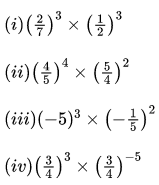Solution: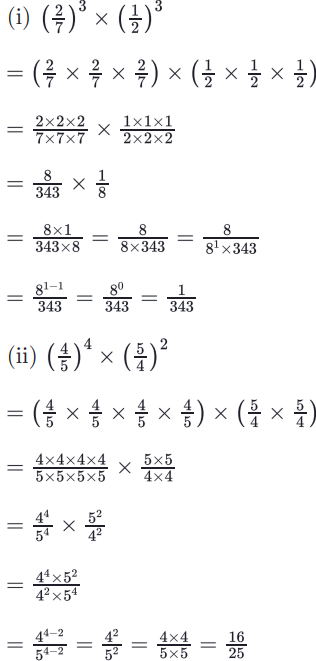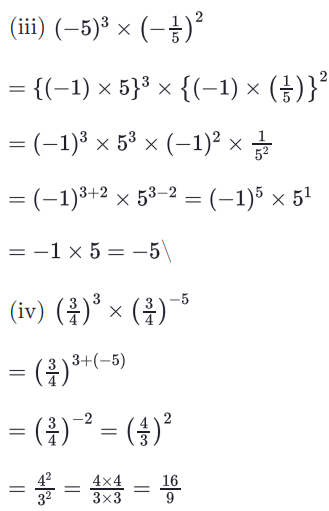Question 2: Find the value of the following.

[i] (-5)3

[ii] [1/2]3

[iii] (-2/3)4

Solution: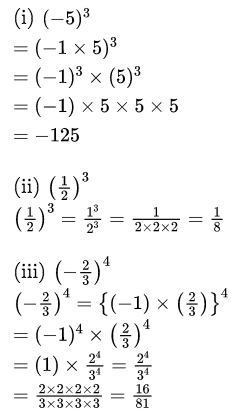Question 3: Using the prime factors, change them into exponential form.

[i] 1/64

[ii] 16/125

[iii] -8/27

[iv] -1/8

[v] -25/49

Solution: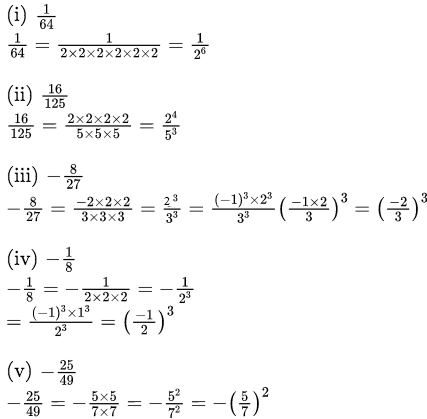Question 4: Simplify the following.

[i] 32 x 33

[ii] [½]2 x [½]3

[iii] [3/2]2 x [3/2]3

[iv] [-½]3 x [-½]4

[v] [⅖]2 x [⅖]3

Solution: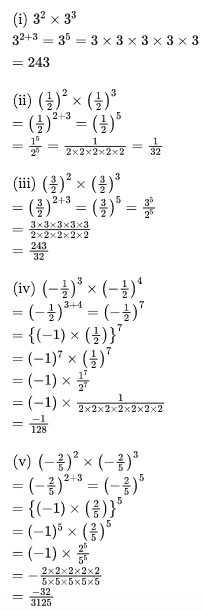Question 5: Answer the following in exponent form.

[i] 45 ÷ 42

[ii] (-5)7 ÷ (-5)4

[iii] [⅔]5 ÷ [⅔]4

[iv] [-⅕]11 ÷ [-⅕]6

Solution: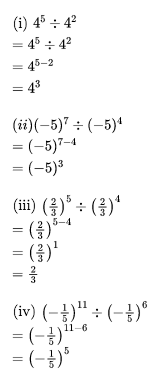Question 6: Find the value of the following.

[i] 3

[ii] 2

[iii] 2

[iv] [-24]2

[v] [(½)2]4

[vi] [(-1/3)3]2

Solution: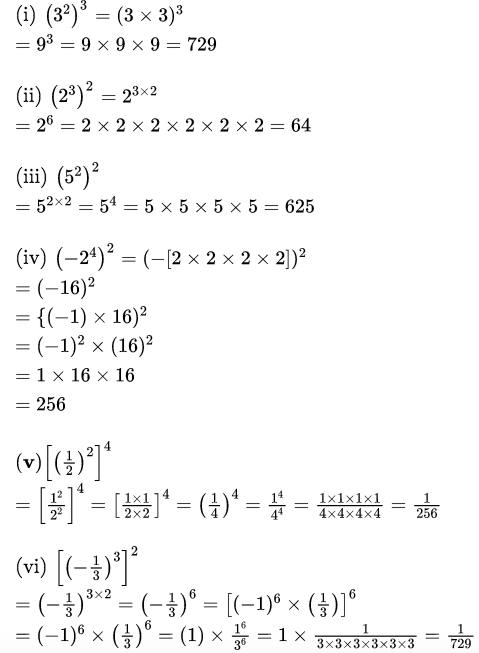Question 7: Solve the following.

[i] 30

[ii] 75-5

[iii] [-2]3-3

[iv] [⅖]2+3-5

[v] 20 x 30

[vi] 20 + 50

[vii] [7/15]0 + [1/7]3-3

Solution: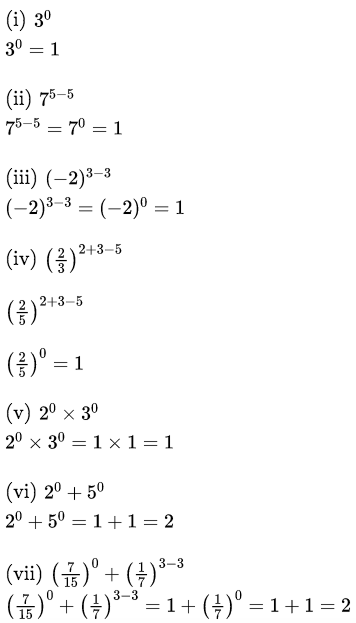Question 8: Change the following into positive exponent numbers.

[i] 2-3

[ii] 3-5

[iii] a-4

[iv] (-2)-5

[v] (-x)-3

[vi]

$$\begin{array}{l}\frac{1}{5^{-3}}\end{array}$$

[vii]

$$\begin{array}{l}\frac{1}{y^{-3}}\end{array}$$

[viii]

$$\begin{array}{l}\frac{1}{[\frac{2}{3}]^{-3}}\end{array}$$

Solution: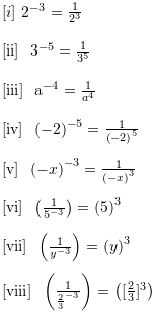Question 9: Simplify the following exponents.

[i] (22 x 33)2

[ii] [15/16]3 ÷ [9/8]2

[iii] [4/9]2 ÷ [28/27]3

[iv] (⅔)2 x (¼)3 x (3/4)2

[v] [52 / 32]2

[vi]

$$\begin{array}{l}\left[\frac{2^2\times 3^2}{2^3\times 6^2}\right]^2\end{array}$$

Solution: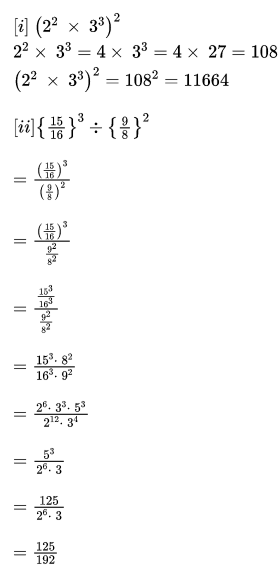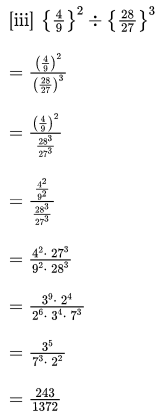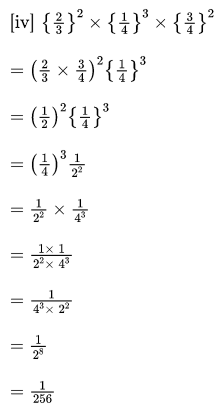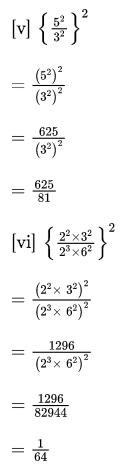Question 10: Find the value of the following.

$$\begin{array}{l}\left[\frac{1}{3}\right]^{-2}+\left[\frac{1}{2}\right]^{-2}+\left[\frac{1}{4}\right]^{-2}\end{array}$$

Solution: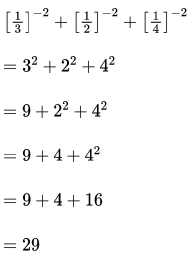RBSE Maths Chapter 3: Exercise 3.2 Textbook Important Questions and Solutions

Question 1: Find the value of x.

[4/3]-4 x [4/3]-5 = [4/3]-3x

Solution:

[4/3][-4]+[-5] = [4/3]-3x

[4/3]-9 = [4/3]-3x

Since the bases are the same, we can equate the powers.

-9 = -3x

x = -9/-3

x = -3

Question 2: Find the value of [3125 x 1296] / [6561 x 1875].

Solution: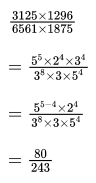RBSE Maths Chapter 3: Exercise 3.3 Textbook Important Questions and Solutions

Question 1: Write the following in standard form.

[i] 128000000

[ii] 0.0005

Solution:

[i] 128000000

= 1.28 x 100000000

= 1.28 x 108

[ii] 0.0005

= 5 / 10000

= 5 / 104

= 5 x 10-4